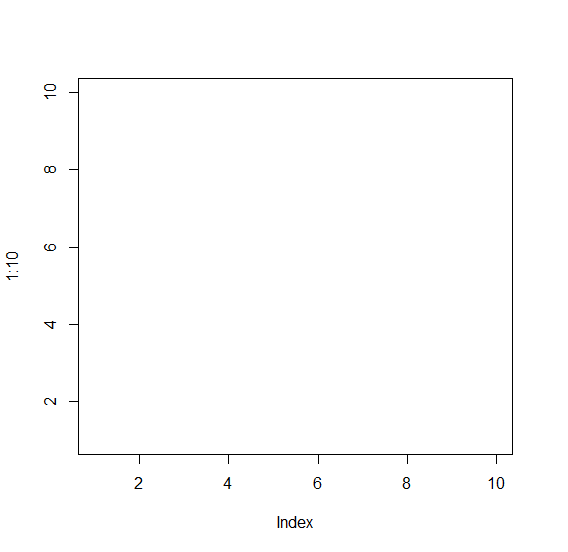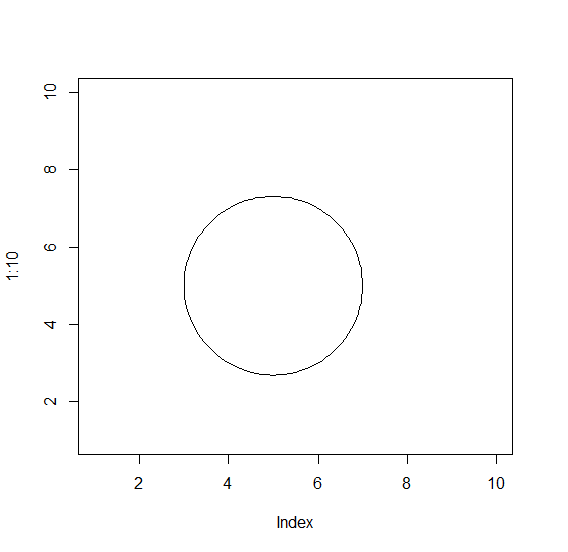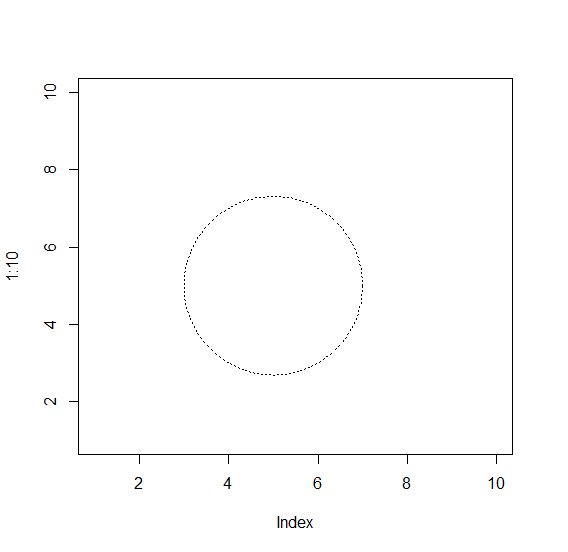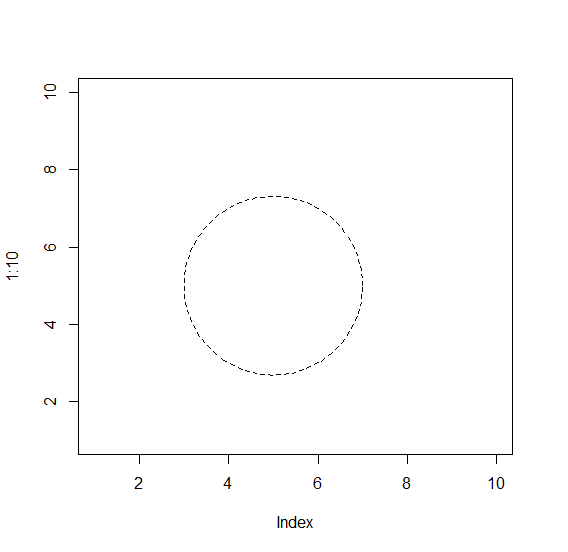# How to change the border style of a circle in R?

We can create a circle in R by using draw.circle function of plotrix package and default border will be like straight lines. If we want to change the border style of a circle then we can use lty argument and set it to different values as shown in the below Examples.

## Example

To change the border style of a circle in R use the following snippet −

plot(1:10,type="n")

## Output

If you execute the above given snippet, it generates the following Output −To change the border style of a circle in R add the following code to the above snippet −

plot(1:10,type="n")
library(plotrix)
draw.circle(5,5,2)


## Output

If you execute all the above given snippets as a single program, it generates the following Output −To change the border style of a circle in R add the following code to the above snippet −

plot(1:10,type="n")
library(plotrix)
draw.circle(5,5,2,lty=9)

## Output

If you execute all the above given snippets as a single program, it generates the following Output −To change the border style of a circle in R add the following code to the above snippet −

plot(1:10,type="n")
library(plotrix)
draw.circle(5,5,2,lty=20)

## Output

If you execute all the above given snippets as a single program, it generates the following Output −